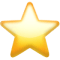Search app# Solve4x Math Equation Solver

(0)
Price
Free
Category
Education & Utilities
Last Update
Dec 18, 2015
View in store

#### Store Performance Index

64%

Store Performance Index shows overall performance of your app on app stores. It is based on combined ASO & reviews metrics.

See more data

## Ratings & Reviews performance for Solve4x Math Equation Solver

Ratings & Reviews performance provides an overview of what users think of your app. Here are the key metrics to help you identify how your app is rated by users and how successful is your review management strategy.

Number of reviews,
total

0

Avg rating,
total0.0

#### Description

2476 chars

Featured in the list "11 Apps That Will Make You Hate Maths a Little Less.." --- gadgets.ndtv.com "no need to struggle with math problems.."-- appPicker.com Camera based Math equation solver Solve4x - The Basic Math & Algebra equations solver app (Level -1) ! NO INTERNET NEEDED TO SOLVE THE EQUATIONS Solves Linear equations in up to 3 variables, quadratic / cubic equations in one variable. You can either type in the equations or Take a photo and scan it. It solves the equations in a Snap ! There is an added function to solve a 2x2 or 3x3 square matrices and find their Inverse and Determinant values. The app is suited for middle and high school students , students can as an example verify their homework. Higher grade students or other academicians can use this app to solve their routine problems and expedite their work. This app is NOT designed to use as a calculator or Pseudo calculator. So if you are trying to use it as as a calculator by entering data as entered in a calculator and then just adding the equate sign along with the variable, then the chances are that the solution will not be correct. As an example, 5 + 8 - 3 * 2 / 7 = x will not work, because the left hand side of this equation is essentially only a calculator function. i.e. the left hand side would have been solved just by using an ordinary calculator without having to resort to add the extra equate sign with the variable on the right hand side. However 8x = 16 will work because this is how the usual equations are in the grade school book. Please note that 2 and 3 variable problems will need corresponding number of equations. Features:- Solves - Linear equations in up to 3 variables, quadratic / cubic equations in one variable, 2x2 / 3x3 matrix. Does NOT solve any other type of equation. This version does not handle parenthesis (brackets), fractions, radicals, complex numbers, trigonometry / logarithmic / calculus / other advanced functions. Photo related - No handwriting recognition. Proper recognition is only in portrait mode (camera upright vertical with Home button down) for printed text. Average size (not too small or too big). Black text on white background appears to work best. Some editing maybe needed to remove any non-equation / junk data. Also depends on lighting. Does not work for front facing camera. Please use the inbuilt Bug reporting tool to report any bugs to us. We are constantly improving this technology to minimize the errors.

User reviews affect conversion to installs and app rating. Featured and helpful reviews are the first to be noticed by users and in case of no response can affect download rate. This is why it is highly recommended to reply to them.#### About Solve4x Math Equation Solver

Developed by Appzest Inc.

Solve4x Math Equation Solver is ranking in   Education & Utilities

Last update was at Dec 18, 2015and the current version is 1.1.

Solve4x Math Equation Solver have a 0a user reviews.

Solve4x Math Equation Solver was released in the App Store. It is developed by Appzest Inc, who have also released the following apps.

Solve4x Math Equation Solver has 0 user reviews.​

Solve4x Math Equation Solver has an average rating of 0.0. ​The latest version of Solve4x Math Equation Solver 1.1​ ​​was ​released on Dec 18, 2015​.